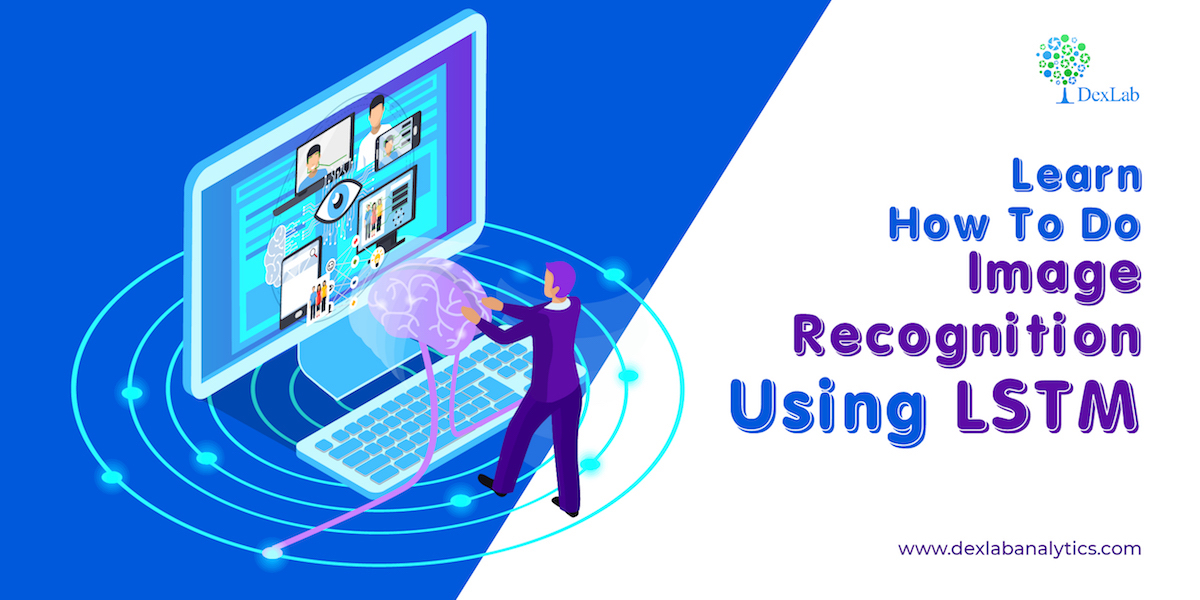Image Recognition Using LSTM Archives - DexLab Analytics | Big Data Hadoop SAS R Analytics Predictive Modeling & Excel VBA

## Learn How To Do Image Recognition Using LSTMThis is a tutorial where we teach you to do image recognition using LSTM. To get to the core you have to understand that how a convolutional neural network perceives the data. In this tutorial the data we have is four-dimensional data, so, you need to convert the dataset accordingly. You can find the tutorial video attached to this blog.

Now suppose there is an image 28 by 28 pixel, if the image is black and white then there would be only one channel. So how will you put the data in CNN, it will be like the number of samples, then followed by the number of rows of the data, then the number of columns, then channels. These are the four values that need to be provided in the input layer, at the very beginning. Now, these values must be converted according to the LSTM. Now the LSTM wants the STF, like the number of samples, time steps like how many time steps back you want to go for making further prediction because LSTM is a sequence generator and the number of features. So, we will be converting the image that is the number of sample 28 by 28 one pixel into one sample of 28 by 28, that’s the only job you have to do and all you need to accomplish this is to prepare the data accordingly.

There will be no mysteries here, in fact, it is a normal neural network LSTM, that anybody can run in a most simple form, and in this tutorial, it is also run in the most simple form there is no complexity involved and only a few epochs will be run.

#### You can find the code sheet you need for this at

Also follow this video that explains the process step by step, so that you can easily grasp how LSTM can be used for the purpose of image recognition. To access more informative tutorial sessions like this follow the DexLab Analytics blog.

.

+91 931 572 5902